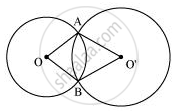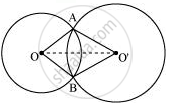# Prove that the line of centres of two intersecting circles subtends equal angles at the two points of intersection. - Mathematics

Prove that the line of centres of two intersecting circles subtends equal angles at the two points of intersection.

#### SolutionLet two circles having their centres as O and O' intersect each other at point A and B respectively. Let us join OO'.In ΔAOO' and BOO',

OA = OB (Radius of circle 1)

O'A = O'B (Radius of circle 2)

OO' = OO' (Common)

ΔAOO' ≅ ΔBOO' (By SSS congruence rule)

∠OAO' = ∠OBO' (By CPCT)

Therefore, line of centres of two intersecting circles subtends equal angles at the two points of intersection.

Is there an error in this question or solution?
Chapter 10: Circles - Exercise 10.6 [Page 186]

#### APPEARS IN

NCERT Class 9 Maths
Chapter 10 Circles
Exercise 10.6 | Q 1 | Page 186

Share